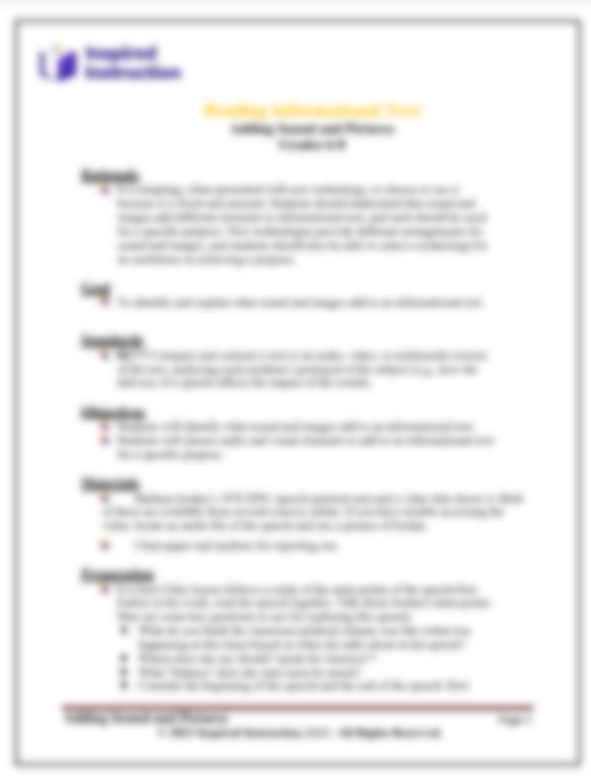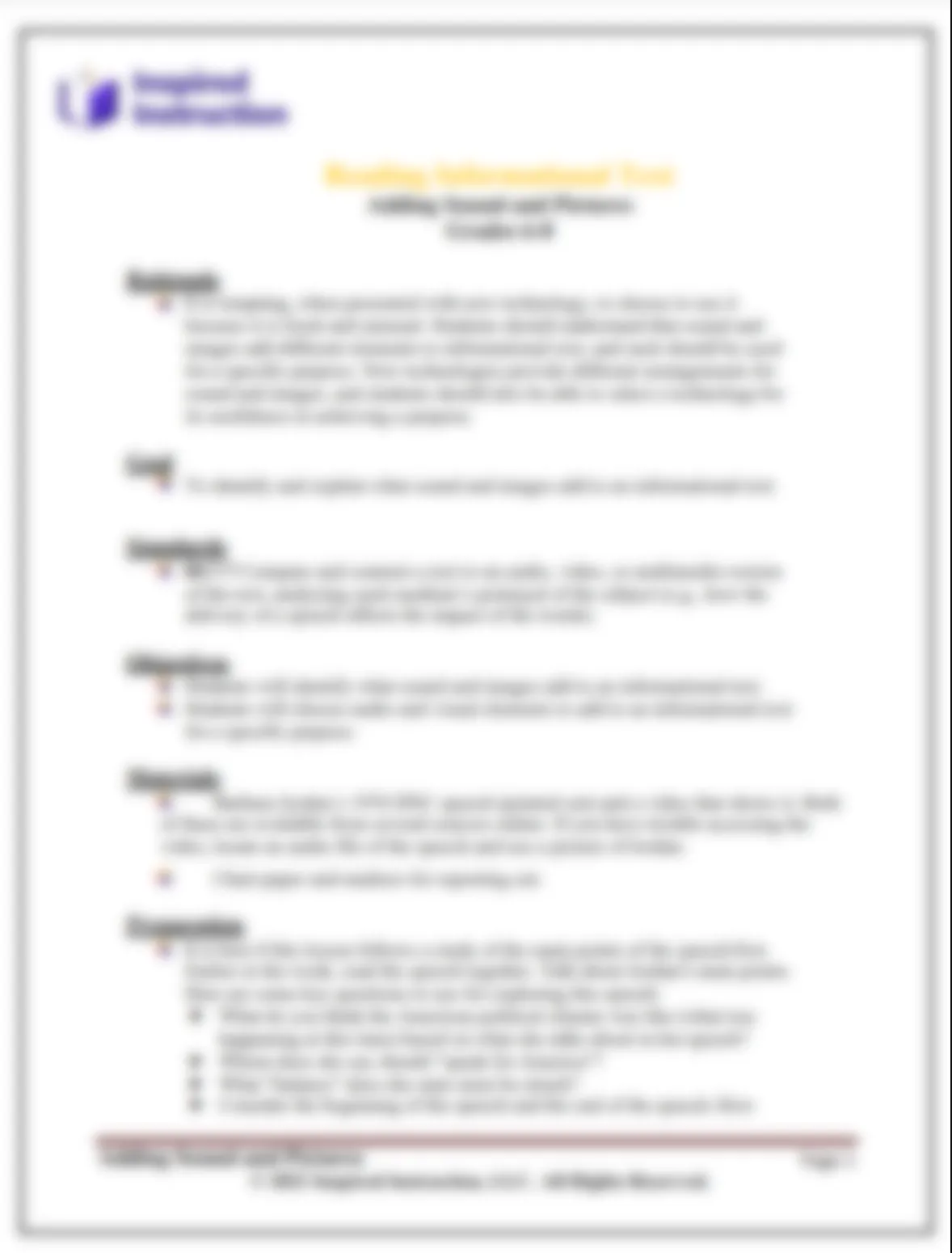# Grade 3 Math, Lesson 6: Constructed-Response Strategies

There are a variety of mathematical models, methods and strategies that students can employ to respond to mathematical items. Many students employ a combination of strategies. During this lesson, students will share and employ strategies, and begin considering how they can most efficiently respond to assessment questions. Students need to explore using different strategies for each style of item they will encounter on the NJSLA. Students need to practice constructed-response problems in which students are providing the solution to the given problem or completing a mathematical model that represents the problem and has missing features.

Subject:
PARCC/NJSLA
Content Type:
Lesson Plans

Grade 3 Math, Lesson 6: Constructed-Response Strategies

Grade 3 Math, Lesson 6: Constructed-Response Strategies

Grade 3 Math, Lesson 6: Constructed-Response Strategies

Grade 3 Math, Lesson 6: Constructed-Response Strategies

Grade 3 Math, Lesson 6: Constructed-Response Strategies

## This content is exclusive to Members## This content is exclusive to Partner members## This content is exclusive to Partner+ membersGrade 3 Math, Lesson 6: Constructed-Response StrategiesSchoolDistrictOtherOops! Something went wrong while submitting the form.

#### Related workshops you may find helpful

NJSLA Mathematics

### Integrating NJSLA into Daily Instruction for Math

NJSLA Mathematics

NJSLA

PARCC/NJSLA

### Grade 5 RST Reading, Lesson 5: Using Context Clues for the Vocabulary EBSR